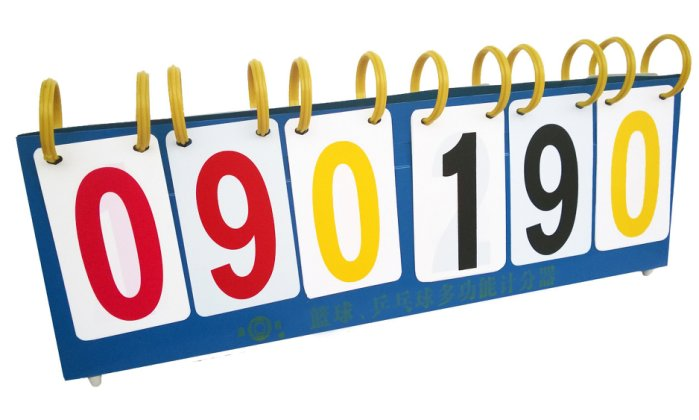「游族杯」上海市高校程序设计邀请赛暨华东师范大学第九届 ECNU Coder 程序设计竞赛 (重现)

A. 足球锦标赛

ECNU 足球锦标赛采用了最传统的计分牌来计分。每进一个球，计分员给对应的队要翻牌，使得计分板上显示的数加一。Input
2
009 009
2
001 003
2
Output
Case 1: 20
Case 2: 2

提示

• 甲方（前者）得 $2$ 分，乙方（后者）得 $0$ 分；甲方要翻 $10 + 1 = 11$ 次。
• 甲方和乙方各得 $1$ 分，共要翻 $10 + 10 = 20$ 次。
• 乙方得 $2$ 分，同样翻 $11$ 次。

NaN